# Overview

The Regression analysis implements a multiple linear regression model. The analysis aims to model the relationship between a dependent series and one or more explanatory series. Several models can be specified within one instance of the analysis. The output consists of the coefficients of the linear model, the predicted series and several statistical indicators. If there is sufficient data after the end of the estimation sample range, forecasts can be calculated.

## Estimation model

The Regression analysis attempts to find the linear combination of a number of explanatory series that best describes a dependent series.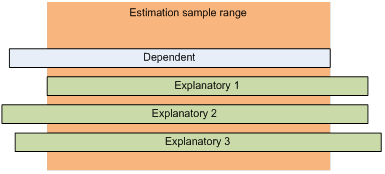The analysis uses the following model:

${\text{y}}_{\text{t}}=\text{α}+{\text{β}}_{\text{1}}\phantom{\rule{0ex}{0ex}}{x}_{\text{t1}}+{\text{β}}_{\text{2}}{x}_{\text{t2}}+{\text{β}}_{\text{3}}{x}_{\text{t3}}+{\text{ϵ}}_{\text{t}}$

where y is the dependent series and x_i are the explanatory series. If the option “No intercept” is selected, then the constant α is not included in the model.

The parameters α and β are estimated by minimizing the sum of the squared residuals ϵ. This is known as Ordinary Least Squares (OLS). The output from the analysis will include the predicted series calculated using the estimated parameters.

The automatic estimation sample range will be the largest range where there is data for all series. You can specify a smaller range if you like to limit the data to be used in the estimation.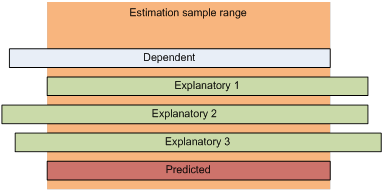### Report

The Regression analysis automatically generates a report, which includes variety of statistical information.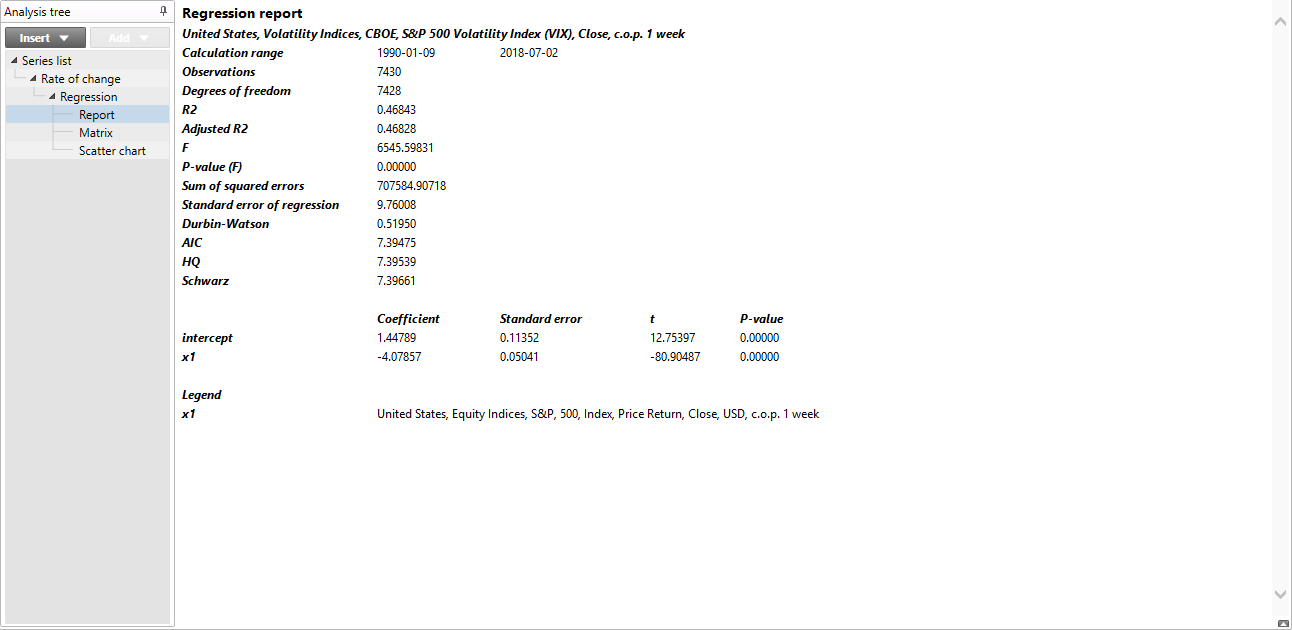#### Calculation range

The calculation range used for the analysis.

#### Observations

The number of observations used in the analysis. This includes all observations in the calculation range where there are values for all series.

#### Degrees of freedom

The number of observations minus the number of dependent series and minus one for the constant parameter.

#### R2

Compares the variance of the estimation with the total variance. The better the result fits the data compared to a simple average, the closer this value is to 1.

#### F

The F-ratio is the ratio of the explained variability and the unexplained variability each divided by the corresponding degrees of freedom. In general, a larger F, indicates a more useful model.

#### P-value (F)

The p-value is the probability of obtaining a value of F that is at least as extreme as the one that was actually observed if the true values of all the coefficients are zero.

#### Sum of squared errors

The sum of the square of the residuals.

#### Standard error of regression

The square root of the sum of squared errors divided by the degrees of freedom. This is an estimate of the standard deviation of residuals.

#### Standard error of forecasts

The square root of the sum of squared forecast residuals divided by the number of residuals.

#### Durbin-Watson

The Durbin-Watson is a test statistic used to detect the presence of autocorrelation in the residuals. The value is in the range 0-4. A value close to 2 means that there is little auto correlation. The result from this test is not useful if any dependent series is included with several lags or if no intercept is included in the model.

#### Information criteria

The information criteria are measures of the expected information loss. A lower value means that more information is captured. This can be used to compare models when the same data is used in the models.

##### AIC

Akaike's information criterion.

##### HQ

Hannan and Quinn's information criterion

##### Schwarz

Schwarz criterion also known as Bayesian information criterion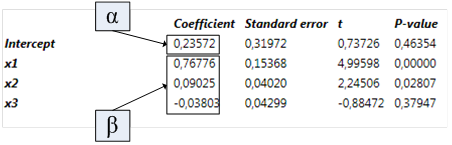#### Coefficient

The estimated parameters

#### Standard error

The standard error of the estimated parameters

#### t

The estimated coefficient divided by the standard error

#### P-value

The p-value is the probability of obtaining a value of t that is at least as extreme as the one that was actually observed if the true value of the coefficient is zero

### Forecast

When there are data for all the explanatory series beyond the estimation sample, we can use the estimated parameters to calculate forecasts. This is done by checking the option “Calculate forecast”. If no end point is specified, the analysis will calculate as many forecasted values as possible. You can specify an end point if you want to limit the length of the forecast.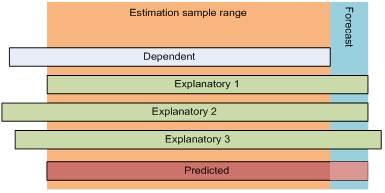### Dynamic forecast

Dynamic forecasting uses the data generated by the model as input to the model to calculate additional forecasts. In this example we have included an explanatory variable that is a lag of the dependent series.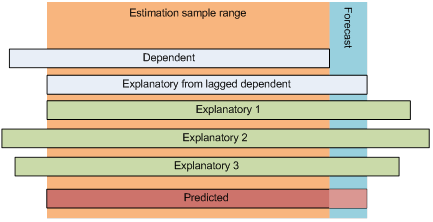In the example, it is the lagged series that limits how far we can calculate the forecast. This allows us to use the predicted data as input to calculate the forecast further. The analysis will only attempt to do this if you select the “Dynamic forecast” option.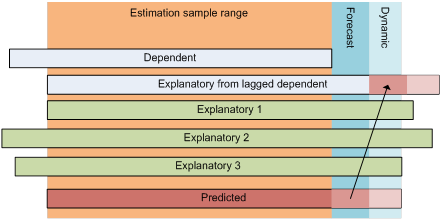There is one special case to be aware of. If all the explanatory series are lagged versions of the dependent series, you can use dynamic forecast on the series infinitely many times. In this case you must specify an end for the forecast since there is no way for the application to know when to stop.

# Settings### Regression models

You can define one or more regression models. Each model has separate settings. When a new model is created, the settings of the current model are duplicated. Models can be renamed and deleted.

### Output dependent series

Select this option to include the dependent series in the output.

### Output explanatory series

Select this option to include the explanatory series in the output.

### Estimation sample range

Specify the limits of the estimation sample range. The default range will be the largest range where there is data for all the series.

### No intercept

When this option is selected, the constant α is omitted from the model and it will be defined as:

${\text{y}}_{\text{t}}={\text{β}}_{\text{1}}\phantom{\rule{0ex}{0ex}}{x}_{\text{t1}}+{\text{β}}_{\text{2}}{x}_{\text{t2}}+{\text{β}}_{\text{3}}{x}_{\text{t3}}+{\text{ϵ}}_{\text{t}}$

### Residuals

When this option is selected a series containing the residuals will be included in the output.

### Residuals for forecasts

If this option is selected, the series of residuals will also contain residuals for the forecasted values. Such residuals can only be calculated when forecasts are calculated and there is an overlap between the forecasts and the dependent series.

### Uncertainty band

By selecting the option Uncertainty band, two additional time series will be calculated. These time series form a band around the predicted values by adding and subtracting a number of standard deviations. The standard deviations is the measurement Standard error of regression, as described in the section Report above.

### Calculate forecasts

Forecasts will be calculated only if this option is selected and there is sufficient data, as explained in the section Forecast above.

### End point

You can limit how far into the future that forecasts will be calculated. If not specified, forecasts will be calculated as far as possible.

In the special case when dynamic forecast is enabled and the model contains only lagged versions of the dependent variable, a limit must be specified.

### Allow dynamic forecast

Allow the use of predicted values of the dependent series when calculating forecasts.

### Confidence band

By selecting the option Confidence band, two additional series will be calculated. These time series form a confidence band around the forecasted values. The band is calculated so that the forecast is within the band with the specified probability assuming that the forecast values are t-distributed.

### Include

Select if you want to include this series in the model.

### Is dependent

Select which series is the dependent series. This must be specified.

### Diff

Available from Macrobond version 1.19

By selecting Diff, the first order differences of the series will be calculated. The result will then be converted back to levels. First order of differences means that the series is transformed to "Change in value" (one observation) while expressing the result in levels.

### Lag to/from and Lag range

Here you specify the lags you would like to include for a specific series. When lagging a series, the values are delayed in time and the series stretches further into the future.

If you for example set “Lag from” to 0 and “Lag to” to 2 three series will be included, one series with no lag, one with a lag of 1 and one series with 2 lags. This will automatically change the lag range to “0 to 2”. You may specify the desired lags using Lag to/from or Lag range, the result will be the same. If you set Lag range to a single digit or set Lag to and Lag from to the same value, a single lagged series will be included.

When lags are specified for the dependent series, the lagged series will be used as explanatory series in the model. The dependent series will always be without lag.

# Examples

Regression model- multiple series

In the Regression analysis, we first defined the variables of the model, by:

• Marking the Industrial Production as dependent variable
• Specifying the lags for the explanatory series (these numbers are based on the Correlation analysis).
• Defining the output we want to have in the chart: dependent series & residuals

We also decided to calculate forecasts. This is possible as all explanatory variables have been lagged, meaning we can calculate forecasts for the shortest number of lags defined, here 2 months.

Regression S&P and VIX scatter chart

In the regression analysis, we checked as output both the dependent and explanatory variables. Both series, as well as the predicted series, will be needed in the Scatter Chart to show one week change in both in indices.